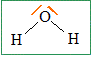Skip to content

# Answer garden math

We know that the two trinagles are right-angled triangles so, 2,5² + a² = 4² 6,25 + a² = 16 16 - 6,25 = a² 9,75 = a² a = √9.75 We know that the quadrilateral is a rectangle so the opposite sides have the same length So 8² + a² = 8,5² 16 + a² = 72,25 72.25 -16 = a² a² = 56,25 a =  √56.25

Posted in Non classé.

# answer of chemestry exercice

1) lewis representation of butanol                                                                        lewis representation of water :2)  M(h2O) = 18g/mol n(h2O) = m(h2O) / M(h2O) n(h2O)=1000/18= 55.55 mol M(C4H10O)= 74 n=m/M =2000/74 =27 MOL In 1kg of butanol, there are 55.5 mol In 2kg of water, there are 27 mol. 3) equation :C3H8+  ...O2--> ....CO2+....H2O the equation complete is : C3H8+  5O2-->3CO2+4H2O

Posted in Group8.```Hello, this is the answer of the first question of the Eiffel tower's problem.
We can't do the others questions because the picture is too little. Can you send
a bigger please ? Thank you.

ABC is isoscele in C.
We call [AH] the leaving height of A.
[AH] divide BA in two equal segments : CH= c×1/2= 97,5×1/2= 48,75 m
[AH] is perpendicular to (AB) so AHC is a right-angle triangle.
We can use the propriety of Pythagore :
HC² + AH² = CA²
48,5² + 800² = CA²
2376,2 + 640000 = CA²
642376,2 = CA²
The 642376,2's square root is equal to 801,5
CA = b = a = 801,5m

Now, we can use trigonometry:
cos(Ĉ) = 800/801,5
Ĉ = cos-1×800/801,5 ≈ 3,5°
Cos(Â) = 48,5/801,5
Â = cos-1×48,5/801,5 ≈ 86,5°```Posted in Group3.

# ENIGMAS !!!

Enigma A A shell is fixed on the hull of a boat, with 3 meters above level of water. Water goes up 2 centimetres per hour. In how long will water touch the shell? Enigma B An Earthworm of 50 G, a millepatte of 30 G and a grasshopper of 20 G want to pass a river. At their disposal, a sheet of tree which can carry to the maximum only 60 G. How will they reach that point? Enigma C A water lily is in a lake. Every day it doubles of size. After 15 days, it makes half of the lake. How long will it put to entirely cover the lake? Enigma D Let us suppose that we have you and me as much money. How much do I have to give you so that you have 10F moreover than me? Enigma E A bookseller buys a book of maths (=D) 70F, sells it 80F, ransoms it 90F, resells it 100F. Which was its benefit?

Posted in Group5.

# answer of the first exercise

1) For this typical french meal :  Yvette ate 6 snails and 8 frogs. Jean-Claude ate 4 snails and 12 frogs. Ginette ate 3 snails and 2 frogs. Gérard ate 5 snails and 3 frogs. And Gertrude ate 4 snails and 3 frogs. At the end of  the evening 22 snails and 28 frogs were eaten. 2) We know that 2 snails cost 3 euros and 1 frog costs 2 euros so : 2 x 28 = 56 euros 2 snails = 3 euros 22 sanils =? --> (22 x 3) / 2 = 33 euros The result is 33 + 56 = 89 euros The family must pay 89 euros for Christmas

Posted in Group9.

# “The eiffel tower” answer

Question 1 :

320=1/2 x 9,81 x T2

320 x 2=9,81 x T2

640=9,81 x T2

T=640/9,81

T=8

The time that will take a man who jump out from the top of the eiffel tower to reach the ground will be of approximately 8s.

Question 2 :

S=9,81 x 602 x ½

S=(9,81 x 3600)/2

S=35316/2

S=17658

If you want to fall in 1 minute, you must be at 17658 m of the ground or 17,658 km.

Question 3 :

I think that it was possible on this planet but ti will be very difficult.

Posted in Group5.

# answers of exercice one of mathematics

The answers of the exercice one is this :
• The equation of the first fonction is f(x)=2X+3
• The equation of the second fonction is f(x)=-2X+3
• The equation of the third fonction is f(x)=(2/5)X-1
• The equation of the fourth fonction is f(x)=-(5/2)X-1
• The equation of the last fonction is f(x)=1
I hope that you have the good solution.

Posted in Group8.

# answer of the exercice about sangaku

To draw this sangaku, you have to draw a semi-circle. Next, draw a triangle in this semi-circle. Next, draw a rectangle in this triangle. With this, you have three right triangle. And to find the circle in the triangle, you have to draw the bisector of this triangle and you have the center of the circle. It was very easy, isn't it ??? For the length, you could take every length.

Posted in Group8.

# Answer of Science assignment

I think I have the answer! But I'm not sure because I don't know potential energy and kinetic energy very much.

For the first question,

m = 225 g = 0.225 kg

g = 9.82 m/s

d = 300 m

potential energy = m x g x d = 0.225 x 9.82 x 300 = 662.85 j

For the second question,

It's the same result because when it hits the ground : potential energy = kinetic energy.

Thank you for your cooperation

Posted in Group1.

# exercise of chemistry

The electronic structure of the atom of azote N is (K)2(L)5.

What is the electronic structure of the atom of phosphore P knowing it is below the atom of azote N in the periodic classification ?

What is the caracteristic of atoms in a same line ?

And in the same column ?

Posted in General information, Group9.